Next: R-BY-C CROZZLE IS      NP-COMPLETE Up: Crozzle: an NP-Complete Problem Previous: Crozzle: an NP-Complete Problem

# INTRODUCTION

The R-by-C Crozzle problem, introduced at the 1996 ACM Symposium on Applied Computing , is a generalization of the Crozzle problem found in The Australian Women's Weekly. A Crozzle is a word puzzle played on a 10x15 grid. Words from a supplied list are placed on the grid subject to the following rules:

1.
Not all of the words need to be placed.
2.
All placed words must fit completely on the grid.
3.
The intersection of two words must be at a shared letter.
4.
No two words may be adjacent (unless the adjacent parts are covered by Rule 3) or placed end-to-end.
5.
The words must form a single connected unit.

A Crozzle is scored as follows: 10 points for each word placed plus points for each letter that appears at the intersection of two words. Letters have the following point values: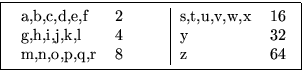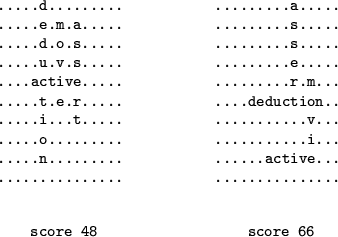Figure 1 shows two solutions to a simple Crozzle. Algorithms for automatically finding good solutions to Crozzles have appeared in the literature [5,3].

The R-by-C Crozzle problem, introduced by Gower and Wilkerson to study the complexity of the original, generalizes the Crozzle problem as follows: in addition to a list of words, an instance of the R-by-C Crozzle problem has as input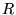and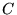, the number of rows and columns in the grid. Thus the original Crozzle problem is R-by-C Crozzle with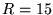and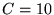.

Gower and Wilkerson argue that R-by-C Crozzle is NP-Hard, but not in NP. Unfortunately this says nothing about the complexity of the original problem as it is possible that restricting R to 15 and C to 10 would place it in NP. Section 2 demonstrates that R-by-C Crozzle is in fact in NP and thus an NP-Complete problem. This implies that the original (more restrictive) Crozzle problem is also NP-Complete.

One technical note: the words supplied as part of a Crozzle are normally English words. There are a finite number of English words; thus, one could, in theory, precompute all possible Crozzle solutions giving a constant time bound to the problem. To study its complexity, we generalize the input to include arbitrary words taken from some finite alphabet.

Next: R-BY-C CROZZLE IS      NP-COMPLETE Up: Crozzle: an NP-Complete Problem Previous: Crozzle: an NP-Complete Problem